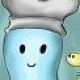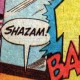# Easy Math Puzzle – Or is it?

How good are you at basic math? Can you solve this simple logic puzzle? Here, give it a go and let me know how long it took you to answer:Got it yet?

It looks easier than it is. The options are presented beautifully to cause maximum mental confusion.

As my dad put it, the answer depends on the answer. If the answer is 60%, it’s 25%. If the answer is 25% it’s 50%. If the answer is 50% it’s 25%. There’s an endless loop with no correct answer.

Don’t lose sleep, I “found” an answer, it was hidden: [edited for clarity]Yes, I photoshopped this. I’m either cheating or engaging in outside-the-box thinking. Sometimes it’s tough to tell the difference.

My preferred set of answers would be:

• A) 25%
• B) 50%
• C) 75%
• D) 50%

Though I’m tempted to throw a “0%” in for good measure…

(Puzzle via PostSecret by way of Spencer of Ask a Mathematician/Ask a Physicist)

[Edited for clarity]

### 19 Responses to Easy Math Puzzle – Or is it?

1.Ike says:

Assuming that one of the answers has to be correct (and given both the format and the context any other assumption by the author would be dishonest), the solution is incorrect.

Please try your puzzle again and see if you can come up with the right answer. Hint: there are only three possibilities.

2.vltava says:

Ike: What is your justification for the assumption that one of the answers has to be correct? (Hint: your assumption is wrong.)

•Ike says:

This is a multiple choice format. So one of the answers has to be correct (as I said, it is dishonest not to include a choice along the lines of none of the above if none of the answers are indeed correct.)

This is a problem of conventions in the English-speaking world, so I’m guessing that this is not your first language. I think I should also point out that your last sentence is considered extremely rude by the usual standards, though that might not be the case in your country.

Now that this is cleared up, there is an unambiguous answer to the problem.

3.Thomas says:

If you pick choice C at random 60% of the time and choice D at random 40% of the time, then the answer is — please wait while I generate a random value — C!

•Jesse Galef says:

Good point! The blackboard never said the random choice had to be evenly distributed between the options listed…

•Randy A MacDonald says:

Not an incorrect solution, but a correct solution to the incorrect problem. Defining ‘random’ also works.

4.Scott says:

The answer is 0%, but only if you *do not* include 0% as an available option.

•Jesse Galef says:

Right, as soon as you include 0% as an option then there’s a chance people will guess it randomly (assuming there’s a non-0 chance of getting it randomly.)

5.David Schreier says:

Questions containing phrases like “this question” or those that refer to the correctness of the as-yet unprovided answer are trouble, and should always be answered “Yes”.

6.skilledchaos says:

It depends on the number of options . 🙂
For four options it’s 25%
For five options it’s 20%
For three options it’s 33%
and so on .
Basically it’s
100%/n
where n is the number of options .
Chance is independant of accuracy or the result itself .So the answer to a chance is fixed . 😀

7.NascentDreaming says:

That does not make sense, because what answer I chose decides logically whether or not my “random answer” is correct. Then the validity of my “random answer” is not random so essentially my answer is not random since the attribute which differentiates my “random answer” from other random answers is a function of my choosing.

8.Patrick says:

Is the intent that the question should be understood as if it read:

“Given a 4 answer multiple choice question in which one of the answers is correct what would the chance of a random choice being the correct answer.”

As it is written I can not see that the question has any meaning.

9.Nick Ergodos says:

The answer is without doubt 25%. As two of the four options says “25%” there is a 50% chance getting it right. However, this is precisely option B which is chosen at random at the desired 25% of all cases. QED.

10.Steve says:

Is ‘answer’ picking a, b, c or d? Or is it picking the ‘content’ of those slots? In which case the two 25%s become significant, and there are only three possible answers.

11.Cliff Mccullar says:

To me (and my tendency to nuke the hell out of questions) this one is very simple and direct if i let it be…but i wont/cant my brain just wont let it. So i think we need to start by over analyzing it(as i think it was intended too, im going to do some of this real quick with the old “if a rooster is on top of your house and lays an egg which way does it fall” question. the “correct” answer being roosters dont lay eggs dummy! or in the case of this question 25% dummy! 4 options chosen truly at random is 25%, it didnt say PICK a random letter and see if your correct but that if you were to do that for any 4 choice multiple choice question at random the likely hood would be 25%. Of course none of this takes into account any number of issues with the question itself which could easily render that incorrect, as this isnt a blind study but a question created by a human mind, which means one of those letters will be dominate(statistically C) but even a person that KNOWS that will still simply subconsciously dominate with another letter unless they sit down and actually ensure that over a 100 question test 25 of the questions are A, B, C and D. Then again the fact that you had to sit down and FORCE it to that means it isnt random and therefore untrue in that context. Then again its been proven again and again that we are incapable of being truly random, heck we cant even write a computer program that gives us true randomness, only the illusion of it. Which makes us go back to the question and realize that it can not be answered because any answer provided by a human is NOT random and therefore incorrect! New millennium problem!

p.s. final point onto my earlier argument that makes the question unsolvable, even for the “simple minded” approach on the 25% answer:note that with A and D being 25% but no option to choose both (remember your answer a what is the percentage for being correct on a 4 choice question NOT to choose randomly.) so since it has given you 2 answers that are correct but only 1 way of answering(traditionally) you can not answer correctly, unless of course you ignore the tradition only circle one answer approach and simply circle 2 answers. note that the question did NOT explicitly deny you that ability.

p.s.s. yeah i know a p.s. is only supposed to be a line or two traditionally, but it felt right to break with tradition in honor of the contents of the p.s. so get over it. 😛

12.Randy MacDonald (@arraymac) says:

Noting that the question does _not_ read, ‘…at random from the answers below,” I choose E) 100%…

13.نواف says:

The answer is 33.3% which is none of these. Its easy if you thinking out of the box.

14.Nico says:

for me it’s “B”. If I’m going to choose an answer at random no matter how many choices there are, there’s a 50% chance that I’m either right or wrong.

15.Dave says:

Reasonable I think to adopt Ike’s convention that one answer must be correct. Then I think it is reasonable to assume prior beliefs that each of the three outcomes (25, 50, 60) are equally likely to be the right answer. Finally, assume that “at random” assumes a well defined probability distribution: let pA, pB, … denote the probability that the random variable is a, b, … respectively.

answer = (pA + pD)*(1/3) + pB*(1/3) + pC*(1/3) = 1/3 since the probabilities must sum to one, which I believe would be Ike’s answer. Holds regardless of probabilities to answer Thomas question.

Note that one could relax the assumption of equal priors to get

answer = (pA + pD)*(Prior(25)) + pB*(Prior(50)) + pC*(1- Prior(25) – Prior(50))

This formula is a legitimate answer, essentially saying the answer depends in an exact way on information not given in the problem.